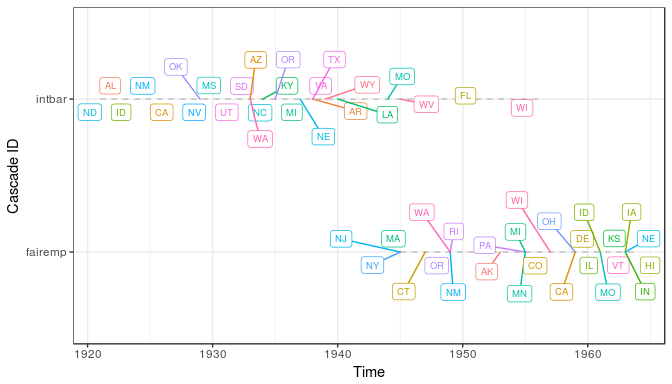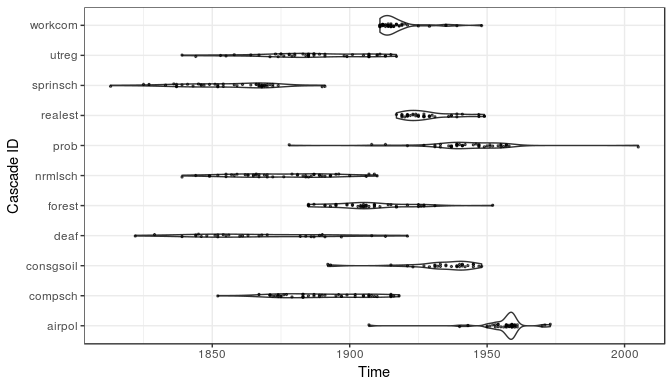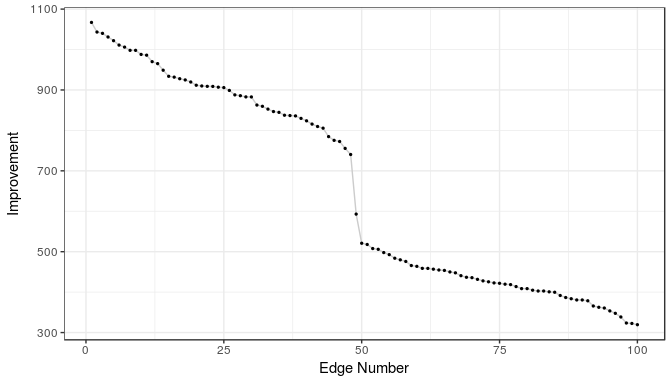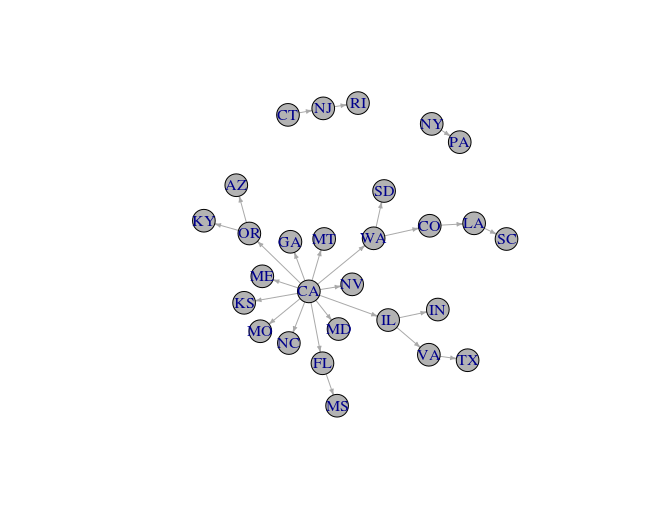# NetworkInference: Inferring Latent Diffusion Networks

## Introduction

This package provides an R implementation of the netinf algorithm created by @gomez2010inferring (see here for more information and the original C++ implementation). Given a set of events that spread between a set of nodes the algorithm infers the most likely stable diffusion network that is underlying the diffusion process.

## Installation

The package can be installed from CRAN:

install.packages("NetworkInference")

The latest development version can be installed from github:

#install.packages(devtools)
devtools::install_github('desmarais-lab/NetworkInference')

## Quick start guide

To get started, get your data into the cascades format required by the netinf function:

library(NetworkInference)

df <- simulate_rnd_cascades(50, n_node = 20)
node_names <- unique(df\$node_name)

# Cast data into cascades object
## From long format

## From wide format
df_matrix <- as.matrix(cascades) ### Create example matrix
cascades <- as_cascade_wide(df_matrix, node_names = node_names)

Then fit the model:

result <- netinf(cascades, trans_mod = "exponential", lambda = 1, n_edges = 5)
## Initializing...
## Inferring Edges...
print(result)
origin_node destination_node improvement
5 16 218.4
20 6 206.7
9 17 190.3
16 4 186.8
3 11 184.9

## Tutorial

This is a quick tutorial to get started with the package. For more detailed information on the algorithm and functionality of the package see the package documentation.

netinf infers the optimal diffusion network from a set of nodes and a number of so called cascades. A cascade is a series of events occurring at a specified time. For this demo we will replicate the analysis presented in @desmarais2015persistent. In this paper Desmarais et al. infer a latent network for policy diffusion based on adoption of 187 policies in the US states. In this case a node in the network is a state, a cascade refers to a specific policy and an event is the adoption of said policy in a state.

### Preparing the Data

The data for @desmarais2015persistent is available in the package:

library(NetworkInference)
# Load the policies dataset (?policies for details).
data(policies)
state_names <- rownames(policies)

In this case the data is stored in a matrix in wide format. Each row corresponds to a state and each column corresponds to a policy. The cell entries indicate the year a state adopted a policy (or NA in case the policy was never adopted). Let’s look at the upper left corner of this matrix:

policies[1:7, 1:7]
equalpay conacchwy soil fish consgsoil airpol forest
CT 1949 1939 1945 1867 NA 1959 1901
ME 1949 1939 1941 1878 NA 1954 1891
MA 1945 1943 1945 1865 NA 1954 1904
NH 1947 1943 1945 1864 NA 1955 1893
RI 1946 1937 1943 1879 NA 1956 1909
VT NA 1955 1939 1867 NA 1959 1904

Most functionality of the NetworkInference package is based on the cascades data format. So before starting with the analysis we have to transform our data to such an object.

policy_cascades <- as_cascade_wide(policies, node_names = state_names)

In this case we used the function as_cascade_wide. If your data is in long format (i.e. each row corresponds to an event rather than a node or unit) you can convert it using the function as_cascade_long.

The cascades data type is basically a list containing all the data stored in three separate objects.

It’s always good practice to visually inspect the data before working with it. The NetworkInference package provides functionality to visualize the cascade data.

The function summary.cascades() provides quick summary statistics on the cascade data:

summary(policy_cascades)
## # cascades: 187
## # nodes: 50
## # nodes in cascades: 50
## # possible edges: 2450
##
## Summary statistics for cascade length and number of ties:
##           length     ties
## Min.    10.00000  1.00000
## 1st Qu. 23.00000 11.00000
## Median  33.00000 19.00000
## Mean    33.13369 20.17647
## 3rd Qu. 46.00000 29.00000
## Max.    50.00000 45.00000

The first four lines provide the number of cascades, the number of nodes in the system, the number of nodes involved in cascades (there might be states that we don’t have diffusion data on, but we still want them represented in the dataset) and the possible number of edges in a potential diffusion network (a diffusion edge between nodes u and v only makes sense if there is at least one cascade in which u experiences an event before v). In this example there are 187 policies and 50 states. Each state is involved in at least one policy cascade and a fully connected diffusion network would have 2450 edges.

It also provides summary statistics on the distribution of the cascade lengths (number of nodes involved in each cascade) and the number of ties in the cascades (two nodes experiencing the same event at the same time). For our example, we can see that the ‘smallest’ policy was adopted by 10 states and the ‘largest’ by all 50 states. From the tie summaries we see that there is at least one policy that was adopted by 45 states in the same year.

The plot() method allows to plot cascades with varying degrees of detail. The argument label_nodes (TRUE/FALSE) provides node labels which require more space but provide more detail. The argument selection allows to pick a subset of cascades to visualize in case there are too many to plot. If label_nodes is set to FALSE each event is depicted by a dot, which allows to visualize more cascades simultaneously.

Let’s first look at the visualization with labels. Here we plot two cascades, selected by their name:

cascade_ids <- colnames(policies)
plot(policy_cascades, label_nodes = TRUE, selection = selection)We can also plot more cascades with less detail:

selection <- cascade_ids[5:15]
plot(policy_cascades, label_nodes = FALSE, selection = selection)This produces a ‘violin plot’ for each cascade with the single diffusion events overplotted as dots. As we already saw in the previous visualization, the policy data has a lot of ties (i.e. many states adopted a policy in the same year) which is indicated by the areas of higher density in the violin plot.

### Inferring the Latent Diffusion Network

The netinf algorithm is implemented in the netinf() function. Besides the data, the function takes three parameters.

trans_mod specifies the transition model, or the parametric model according to which the times between diffusion events are distributed. Currently two distributions are available, the exponential and the Rayleigh distribution. For this example we choose the exponential density.

lambda is the scale parameter for the respective distribution.

n_edges specifies how many edges should be inferred. Best practice is to choose a high number of edges first and then look for a drop-off in gained model fit for each added edge. Then we can rerun the algorithm with a lower number of edges. See @gomez2010inferring and @desmarais2015persistent for guidance on choosing this parameter. The number of inferred edges has to be lower than the maximum number of possible edges. An edge u->v is only possible if in at least one cascade u experiences an event before v. This means, that the maximum number of edges depends on the data. The function count_possible_edges() allows us to compute the maximum number of edges in advance:

npe <- count_possible_edges(cascades)
npe
##  380

Let’s run the algorithm with a larger number of edges to see where the improvement drops off significantly:

results <- netinf(policy_cascades, trans_mod = "exponential", n_edges = 100,
lambda = 1)
## Initializing...
## Inferring Edges...

Let’s take a look at the output of the algorithm. The output is a dataframe containing the inferred latent network in the form of an edgelist:

head(results)
origin_node destination_node improvement
VA TX 1067
CA NC 1043
CA IL 1040
OR KY 1031
WA CO 1022
CA MD 1011

Each row corresponds to a directed edge. The first column indicates the origin node, the second the destination node. The third column displays the gain in model fit from each added edge. Note that the best fitting network would be a fully connected graph, i.e. a diffusion edge between all nodes. However, since we want to infer a sparse network, a model that captures the important diffusion pathways we need to regularize by constraining the number of edges in the network. In order to find a good cutoff, it is good to visualize the gain to check if we can find a sudden drop-off. There is a generic plot method to inspect the results. If more tweaking is required, the results are a dataframe so it should be easy for the more experienced users to make your own plot. With type = "improvement" the improvement from each edge can be plotted.

plot(results, type = "improvement")After inspecting the improvements, the model can be re-run with the desired number of edges. We choose (arbitrarily) 25 here:

diffusion_network <- netinf(policy_cascades, trans_mod = "exponential",
n_edges = 25, lambda = 1)
## Initializing...
## Inferring Edges...

In order to produce a quick visualization of the resulting diffusion network we can use the plot method again, this time with type = "network". Note that in order to use this functionality the igraph package has to be installed.

#install.packages('igraph')
# For this functionality the igraph package has to be installed
# This code is only executed if the package is found:
if(requireNamespace("igraph", quietly = TRUE)) {
plot(diffusion_network, type = "network")
}If additional tweaking of the plot is desired, the network can be visualized using igraph explicitly. We refer you you to the igraph documentation for details on how to customize the plot.

if(requireNamespace("igraph", quietly = TRUE)) {
library(igraph)
g <- graph_from_data_frame(d = results[, 1:2])
plot(g, edge.arrow.size=.3, vertex.color = "grey70")
}